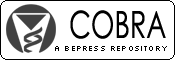## COBRA Preprint Series

#### Abstract

Nonparametric estimation of the probability density function of a random variable measured with error is considered to be a difficult problem, in the sense that depending on the measurement error prop- erty, the estimation rate can be as slow as the logarithm of the sample size. Likewise, nonparametric estimation of the regression function with errors in the covariate suffers the same possibly slow rate. The traditional methods for both problems are based on deconvolution, where the slow convergence rate is caused by the quick convergence to zero of the Fourier transform of the measurement error density, which, unfortunately, appears in the denominators during the construction of these methods. Using a completely different approach of spline-assisted semiparametric methods, we are able to construct nonparametric estimators of both density functions and regression mean functions that achieve the same nonparametric convergence rate as in the error free case. Other than requiring the error-prone variable distribution to be compactly supported, our assumptions are not stronger than in the classical deconvolution literatures. The performance of these methods are demonstrated through some simulations and a data example.

#### Disciplines

Biostatistics | Environmental Public Health

COinS